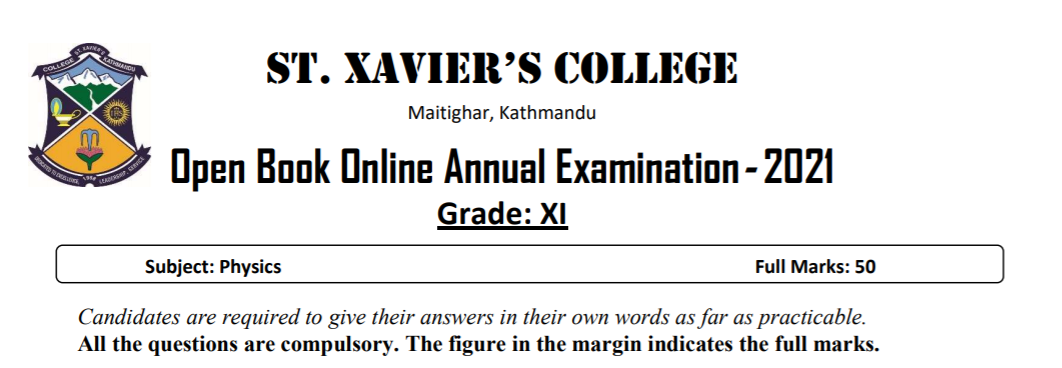Physics Open Book Exam1. The graph below shows the variation of the temperature of 200 g of lead as it is heated at a steady rate.a) Use the graph to state the melting point of lead. 
b) Explain why the graph is a straight line at the start. 
c) Explain what happens to the energy supplied to the lead as it melts at a constant temperature. 
d) The initial temperature of the lead is 0°C. Use the graph to determine the total energy supplied to the lead just to melt.  (The specific heat capacity of lead is 130 J kg−1 K−1.)

2.a) A nucleus of an atom of francium (Fr) contains 87 protons and 133 neutrons. Write down the notation for this nuclide. 
b) If 200 MeV of energy is released in the fission of one nucleus of 92U 235, how many nuclei must fission per second to produce a power of 1 kW? 
c) What is the advantage of doping in semiconductor? What are the factors affecting conductivity of P-type and N-type semiconductors? [1+1]

3. State Newton’s second law of motion. 
a) A delivery company suggests using a remote-controlled aircraft to drop a parcel into the garden of a customer. When the aircraft is vertically above point P on the ground, it releases the parcel with a velocity that is horizontal and of magnitude 5.4 m s–1. The path of the parcel is shown in Figure 1.The parcel takes a time of 0.81 s after its release to reach point Q on the horizontal ground. Assume air resistance is negligible.
(i) On Figure 1draw an arrow from point X to show the direction of the acceleration of the parcel when it is at that point. 
(ii) Determine the height h of the parcel above the ground when it is released 
(iii) Calculate the horizontal distance d between points P and Q. 

b) Another parcel is accidentally released from rest by a different aircraft when it is hovering at a great height above the ground. Air resistance is now significant.
(i) Draw arrows to show the directions of the forces acting on the parcel as it falls vertically downwards. Label each arrow with the name of the force.4.a) What happens to the focal length of lens when it is immersed into water? 
b) A diverging lens and an object are positioned as shown in the Figure 2. Which of the rays A, B, C and D could emanate from point Q at the top of the object and why?Figure 2

5.A uniform electric field between between two parallel conducting plates 1.0 cm apart and are connected to a 100 V battery as shown in the Figure 3, the field is vertically upward and has magnitude 𝐸 = 1.00 × 104 N/C ,
a) If a electron( charge 𝑒 = −1.60 × 10-9 C, 𝑚 = 9.11 × 10-31 kg) is released from rest at the upper plate, what is its acceleration? 
b) What speed and kinetic energy does it acquire while travelling 1. 0 cm to the lower plate?6. How much work must be done to lift an artificial satellite of mass m from the surface of earth of mass M, radius R and put in a circular orbit with radius equal to twice the earth’s radius? 
a) What is the sense of rotation of a geostationary Satellite? How does the GPS system work? [1+2]
b) On a planet whose size is the same and mass 4 times as that of earth, find the amount of work done to lift 6 kg of mass vertically upward through a distance of 3m on the planet. The value of g on the surface of earth is 10 m/s2 . 

7. Metal wire is used to connect a power supply to a lamp. The wire has a total resistance of 3.4 Ω and the metal has a resistivity of 2.6 × 10–8Ω m. The total length of the wire is 59 m.
a) Show that the wire has a cross-sectional area of 4.5 × 10–7 m2 . 
b) The potential difference across the total length of wire is 1.8 V. Calculate the current in the wire. 
c) The number density of the free electrons in the wire is 6.1 × 1028 m–3. Calculate the average drift velocity of the free electrons in the wire. 

8. State two conditions for an object to be in equilibrium.  A sphere of weight 2.4 N is suspended by a wire from a fixed-point P. A horizontal string is used to hold the sphere in equilibrium with the wire at an angle of 53° to the horizontal, as shown in Figure 4a) Calculate
i. Tension T on the wire 
ii. The force exerted by the string on the sphere 
iii. The wire has a circular cross-section of diameter 0.50 mm. Determine the stress in the wire. 

*****